## 2012年5月29日 星期二

### jQuery 練習題：三層式連動下拉選單（無後端整合）

#### 臺北市政府公開資料平台#### 功能介紹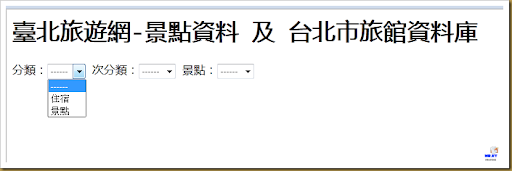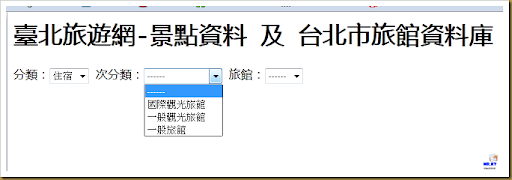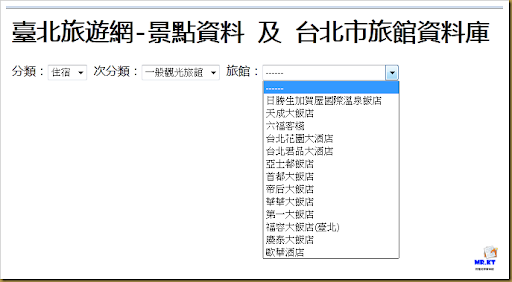#### 引用檔案

jQuery-1.7.2

jQuery XML to JSON Plugin v1.1

http://www.fyneworks.com/jquery/xml-to-json/

LINQ to JavaScript (JSLINQ) v2.2

http://jslinq.codeplex.com/

#### category.txt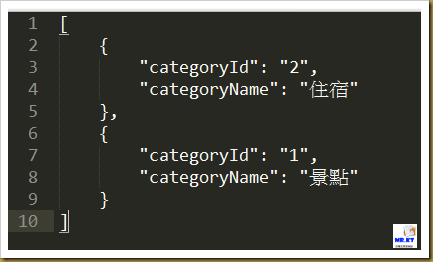SubCategory_01.txt  - 景點次分類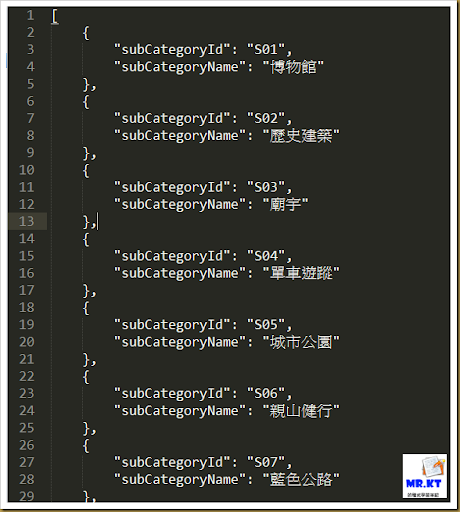SubCategory_02.txt - 住宿次分類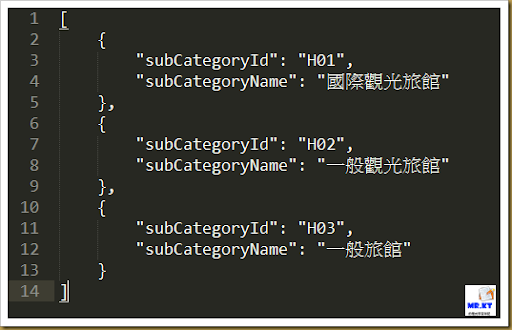#### HTML Code

HTML 的部份相當簡單，

`<h1>臺北旅遊網-景點資料 及 台北市旅館資料庫</h1>`
` `
`<div id="DropDownList">`
`    分類：<select id="SelectCategory"></select> `
`    次分類：<select id="SelectSubCategory"></select> `
`    <label id="LabelViewpoint"></label>：<select id="SelectViewpoint"></select> `
`</div>`

#### Include Javascript files

`<script type="text/javascript" src="scripts/jquery-1.7.2.min.js"></script>`
`<script type="text/javascript" src="scripts/jquery.xml2json.js"></script>`
`<script type="text/javascript" src="scripts/JSLINQ.js"></script>`

Javascript Code

` `
`    var jsonScenery = [];`
`    var jsonHotel = [];    `
` `
` `
`    \$(document).ready(function()`
`    {`
`        Page_Init();`
` `
`    });`
` `
`    function Page_Init()`
`    {`
`        \$.getJSON('data/category.txt', function(data) `
`        {`
`            \$('#SelectCategory').empty().append(\$('<option></option>').val('').text('------'));`
` `
`            \$.each(data, function (i, item)`
`            {`
`                \$('#SelectCategory').append(\$('<option></option>').val(item.categoryId).text(item.categoryName));`
`            });                      `
`        }); `
` `
`        \$('#SelectSubCategory').empty().append(\$('<option></option>').val('').text('------'));`
`        \$('#SelectViewpoint').empty().append(\$('<option></option>').val('').text('------'));    `
`        \$('#LabelViewpoint').text('景點');`
` `
`        \$('#SelectCategory').change(function(){`
`            ChangeCategory();`
`        });`
` `
`        \$('#SelectSubCategory').change(function(){`
`            ChangeSubCategory();`
`        });                `
` `
`        GetSceneryJsonData();`
`        GetHotelJsonData();`
`    }`
` `
`    function GetSceneryJsonData()`
`    {`
`        \$.get('data/scenery.xml', function(xml) `
`        {`
`            jsonScenery = \$.xml2json(xml);`
`        });`
`        return jsonScenery;`
`    }    `
` `
`    function GetHotelJsonData()`
`    {`
`        \$.get('data/hotel.xml', function(xml) `
`        {`
`            jsonHotel = \$.xml2json(xml);`
`        });`
`        return jsonHotel;`
`    }        `
` `
` `

`    function ChangeCategory()`
`    {`
`        //變動第一個下拉選單`
` `
`        \$('#SelectSubCategory').empty().append(\$('<option></option>').val('').text('------'));`
`        \$('#SelectViewpoint').empty().append(\$('<option></option>').val('').text('------'));            `
` `
`        var categoryId = \$.trim(\$('#SelectCategory option:selected').val());`
`        if(categoryId.length != 0)`
`        {`
`            \$.getJSON('data/SubCategory_0'+ categoryId +'.txt', function(data)`
`            {`
`                \$.each(data, function(i, item){`
`                    \$('#SelectSubCategory').append(\$('<option></option>').val(item.subCategoryId).text(item.subCategoryName));`
`                });`
`            });`
` `
` `
`            if(categoryId == '1')`
`            {`
`                \$('#LabelViewpoint').text("景點");`
`            }`
`            else`
`            {`
`                \$('#LabelViewpoint').text("旅館");`
`            }                    `
`        }`
`    }`

`    function ChangeSubCategory()`
`    {`
`        //變動第二個下拉選單`
` `
`        \$('#SelectViewpoint').empty().append(\$('<option></option>').val('').text('------'));`
` `
`        var categoryId = \$.trim(\$('#SelectCategory option:selected').val());`
`        var categoryName = \$.trim(\$('#SelectCategory option:selected').text());`
`        var subCategoryName = \$.trim(\$('#SelectSubCategory option:selected').text());`
`        `
`        if(categoryId == '1')`
`        {`
`            //景點`
`            var result = new JSLINQ(this.jsonScenery.Section)`
`                .Where(function (item) { return item.CAT1 == categoryName && item.CAT2 == subCategoryName; })`
`                .Select(function (item) { return item; }).ToArray();`
` `
`            \$.each(result, function(i, item)`
`            {`
`                \$('#SelectViewpoint').append(\$('<option></option>').val(item["SERIAL_NO"]).text(item["stitle"]));`
`            });`
`        }`
`        else if(categoryId == '2')`
`        {`
`            //住宿`
`            var result = new JSLINQ(this.jsonHotel.Section)`
`                .Where(function (item) { return item.CAT1 == categoryName && item.CAT2 == subCategoryName; })`
`                .Select(function (item) { return item; }).ToArray();`
` `
`            \$.each(result, function(i, item)`
`            {`
`             \$('#SelectViewpoint').append(\$('<option></option>').val(item["SERIAL_NO"]).text(item["stitle"]));`
`            });`
`        }`
`    };`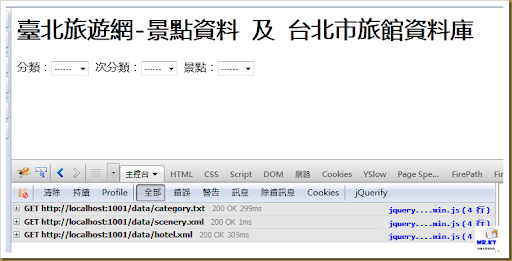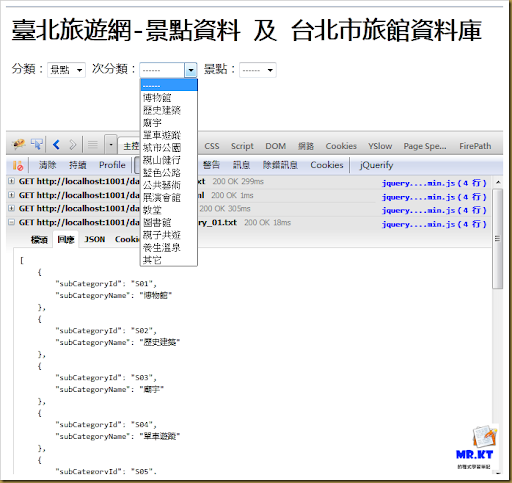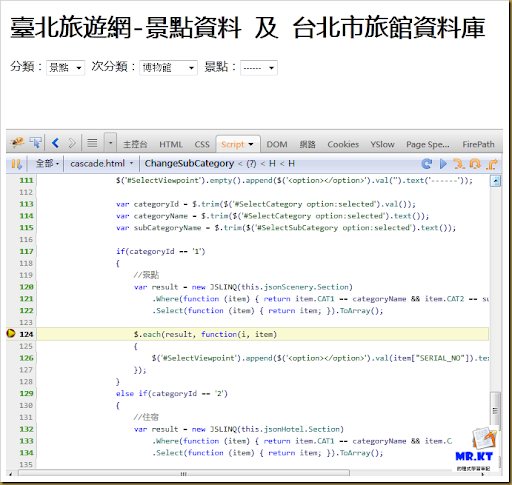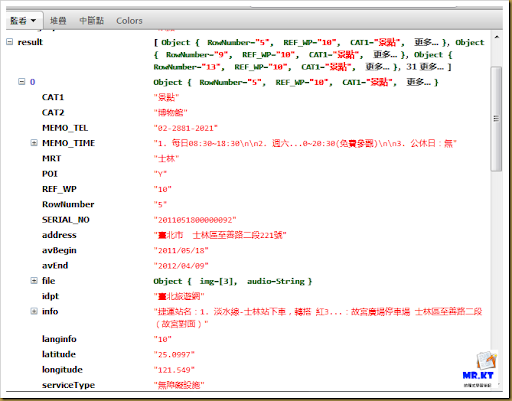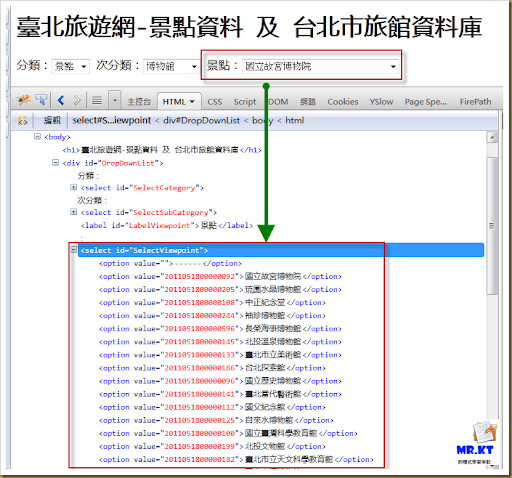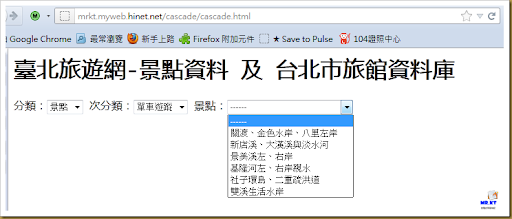`<html>`
`    <head>`
`        <meta http-equiv="Content-Type" content="text/html; charset=UTF-8" />`
`    </head>`
`    <body>`
`        <h1>臺北旅遊網-景點資料 及 台北市旅館資料庫</h1>`
` `
`        <div id="DropDownList">`
`            分類：<select id="SelectCategory"></select> `
`            次分類：<select id="SelectSubCategory"></select> `
`            <label id="LabelViewpoint"></label>：<select id="SelectViewpoint"></select> `
`        </div>`
` `
` `
`        <div id="map" style="width:800px; height:600px"></div>`
`    `
`        <script type="text/javascript" src="scripts/jquery-1.7.2.min.js"></script>`
`        <script type="text/javascript" src="scripts/jquery.xml2json.js"></script>`
`        <script type="text/javascript" src="scripts/JSLINQ.js"></script>`
`        <script type="text/javascript">`
`        <!--`
`            var jsonScenery = [];`
`            var jsonHotel = [];    `
` `
` `
`            \$(document).ready(function()`
`            {`
`                Page_Init();`
`            });`
` `
`            function Page_Init()`
`            {`
`                var jsonData =`
`                [`
`                    {`
`                        "categoryId": "2",`
`                        "categoryName": "住宿"`
`                    },`
`                    {`
`                        "categoryId": "1",`
`                        "categoryName": "景點"`
`                    }`
`                ];`
` `
`                \$('#SelectCategory').empty().append(\$('<option></option>').val('').text('------'));`
` `
`                \$.each(jsonData, function (i, item)`
`                {`
`                    \$('#SelectCategory').append(\$('<option></option>').val(item.categoryId).text(item.categoryName));`
`                });    `
` `
`                \$('#SelectSubCategory').empty().append(\$('<option></option>').val('').text('------'));`
`                \$('#SelectViewpoint').empty().append(\$('<option></option>').val('').text('------'));    `
`                \$('#LabelViewpoint').text('景點');`
` `
`                \$('#SelectCategory').change(function(){`
`                    ChangeCategory();`
`                });`
` `
`                \$('#SelectSubCategory').change(function(){`
`                    ChangeSubCategory();`
`                });                `
` `
`                GetSceneryJsonData();`
`                GetHotelJsonData();`
`            }`
` `
`            function GetSceneryJsonData()`
`            {`
`                \$.get('data/scenery.xml', function(xml) `
`                {`
`                    jsonScenery = \$.xml2json(xml);`
`                });`
`                return jsonScenery;`
`            }    `
` `
`            function GetHotelJsonData()`
`            {`
`                \$.get('data/hotel.xml', function(xml) `
`                {`
`                    jsonHotel = \$.xml2json(xml);`
`                });`
`                return jsonHotel;`
`            }`
` `
`            function ChangeCategory()`
`            {`
`                //變動第一個下拉選單`
` `
`                \$('#SelectSubCategory').empty().append(\$('<option></option>').val('').text('------'));`
`                \$('#SelectViewpoint').empty().append(\$('<option></option>').val('').text('------'));            `
` `
`                var categoryId = \$.trim(\$('#SelectCategory option:selected').val());`
`                `
`                var jsonData = [];`
`                `
`                if(categoryId == '1')`
`                {`
`                    jsonData =`
`                    [`
`                        {`
`                            "subCategoryId": "S01",`
`                            "subCategoryName": "博物館"`
`                        },`
`                        {`
`                            "subCategoryId": "S02",`
`                            "subCategoryName": "歷史建築"`
`                        },`
`                        {`
`                            "subCategoryId": "S03",`
`                            "subCategoryName": "廟宇"`
`                        },`
`                        {`
`                            "subCategoryId": "S04",`
`                            "subCategoryName": "單車遊蹤"`
`                        },`
`                        {`
`                            "subCategoryId": "S05",`
`                            "subCategoryName": "城市公園"`
`                        },`
`                        {`
`                            "subCategoryId": "S06",`
`                            "subCategoryName": "親山健行"`
`                        },`
`                        {`
`                            "subCategoryId": "S07",`
`                            "subCategoryName": "藍色公路"`
`                        },`
`                        {`
`                            "subCategoryId": "S08",`
`                            "subCategoryName": "公共藝術"`
`                        },`
`                        {`
`                            "subCategoryId": "S09",`
`                            "subCategoryName": "展演會館"`
`                        },`
`                        {`
`                            "subCategoryId": "S10",`
`                            "subCategoryName": "教堂"`
`                        },`
`                        {`
`                            "subCategoryId": "S11",`
`                            "subCategoryName": "圖書館"`
`                        },`
`                        {`
`                            "subCategoryId": "S12",`
`                            "subCategoryName": "親子共遊"`
`                        },`
`                        {`
`                            "subCategoryId": "S13",`
`                            "subCategoryName": "養生溫泉"`
`                        },`
`                        {`
`                            "subCategoryId": "S14",`
`                            "subCategoryName": "其它"`
`                        }`
`                    ];`
`                }`
`                else if(categoryId == '2')`
`                {`
`                    jsonData =`
`                    [`
`                        {`
`                            "subCategoryId": "H01",`
`                            "subCategoryName": "國際觀光旅館"`
`                        },`
`                        {`
`                            "subCategoryId": "H02",`
`                            "subCategoryName": "一般觀光旅館"`
`                        },`
`                        {`
`                            "subCategoryId": "H03",`
`                            "subCategoryName": "一般旅館"`
`                        }`
`                    ];                `
`                }`
`                `
`                `
`                if(categoryId.length != 0)`
`                {`
`                    \$.each(jsonData , function(i, item){`
`                        \$('#SelectSubCategory').append(\$('<option></option>').val(item.subCategoryId).text(item.subCategoryName));`
`                    });`
` `
` `
`                    if(categoryId == '1')`
`                    {`
`                        \$('#LabelViewpoint').text("景點");`
`                    }`
`                    else`
`                    {`
`                        \$('#LabelViewpoint').text("旅館");`
`                    }                    `
`                }`
`            }`
` `
`            function ChangeSubCategory()`
`            {`
`                //變動第二個下拉選單`
` `
`                \$('#SelectViewpoint').empty().append(\$('<option></option>').val('').text('------'));`
` `
`                var categoryId = \$.trim(\$('#SelectCategory option:selected').val());`
`                var categoryName = \$.trim(\$('#SelectCategory option:selected').text());`
`                var subCategoryName = \$.trim(\$('#SelectSubCategory option:selected').text());`
`                `
`                if(categoryId == '1')`
`                {`
`                    //景點`
`                    var result = new JSLINQ(this.jsonScenery.Section)`
`                        .Where(function (item) { return item.CAT1 == categoryName && item.CAT2 == subCategoryName; })`
`                        .Select(function (item) { return item; }).ToArray();`
` `
`                    \$.each(result, function(i, item)`
`                    {`
`                        \$('#SelectViewpoint').append(\$('<option></option>').val(item["SERIAL_NO"]).text(item["stitle"]));`
`                    });`
`                }`
`                else if(categoryId == '2')`
`                {`
`                    //住宿`
`                    var result = new JSLINQ(this.jsonHotel.Section)`
`                        .Where(function (item) { return item.CAT1 == categoryName && item.CAT2 == subCategoryName; })`
`                        .Select(function (item) { return item; }).ToArray();`
` `
`                    \$.each(result, function(i, item)`
`                    {`
`                     \$('#SelectViewpoint').append(\$('<option></option>').val(item["SERIAL_NO"]).text(item["stitle"]));`
`                    });`
`                }`
`            };`
`        -->`
`        </script>`
`        `
`    </body>`
`</html>`

1. 對 jQuery 不熟
2. 不知道該用下拉選單的哪個事件觸發
3. 觸發 change 事件後不知道怎麼載入資料
4. 不知道怎麼做來源資料（給下拉選單建立 option 之用）
5. ASP.NET WebForm 開發人員習慣用 UpdatePanel 而對 jQuery, JSON, Javascript 完全不認識

http://www.fyneworks.com/jquery/xml-to-json/#tab-Examples

#### 10 則留言:

1.感謝教學!正好在研究相關的部分，看了這篇有很大的幫助!謝謝~

1.感謝你的回應，能對你有幫助是我的榮幸~

2.大大可以請教一下
如果選單選完,下方想要跳出對應的＜div資料
應該怎麼改寫?
我是美術設計人員><html ok....但script完全不行
最近在做一個靜態網站...剛好要用這個範例(前端搜尋區域)
但我連動選單選完後,下方想出現對應的門市地點
:'(
希望大大能看見啊.....

1.Hello 你好,
有關選完下拉選單的選項之後要把相應的資料給顯示到某一個 ID 的 div 容器下，
我建議你可以參考 jQuery 的官方 API 文件，如下：
http://api.jquery.com/html/
http://api.jquery.com/text/

3.路過，感謝您的資訊。雖然還沒學會，但分享資訊是偉大的

1.4.不好意思打擾了 我目前使用過了三個版本的JSLINQ
在2.10版的 在程式執行時他會顯示JSLINQ.js裡迴圈式子的length有問題
在2.2.2.0 跟2.2.0.0版本的 他在var result = new JSLINQ(this.jsonScenery.Section) 說找不到JSLINQ
於是我把名稱從JSLINQ改成linq.js(2.2.2.0版本的檔案)裡面的Enumerable
他會變成.Select(function (item) { return item; }).ToArray();
這行 .Select找不到
雖小弟才疏學淺 但也是看了程式碼很久都還是沒辦法找到解答...
還請版主不吝指教 感恩

1.因為我不清楚你所使用的資料內容與格式為何，因為我這邊所執行的都是正常（JSLINQ.js），
而有出現問題的應該是使用 IE 瀏覽器（這個請記得在問問題的時候，請務必把你的測試背景資料給說清楚），
然後是直接執行檔案系統下的 html 檔案才會發生，
我會建議，在前端的 javascript 可以不必使用 JSLINQ.js 或 linq.js 這些套件，
我之所以會用，這是因為寫文章的當時正在 try 前端這些類似 .NET 的 LINQ 套件功能，
其實這種過濾條件取得資料的功能，使用基本的 javascript 或 jQuery 也是可以做到，
如果對於 .NET 的 LINQ 並不了解，或是也根本不會用到 .NET 的技術，
那麼就不要管 JSLINQ.js 了，就用最基本的方式來處理，
而至於怎麼改用所謂的最基本的 javascript 或 jquery 的方式來......
我想我就不提供做法，就讓大家自己去練習吧（自己的功課自己寫）

5.您好:
真的非常抱歉,想請問要如何連結至指定的檔案呢?是否有完整的語法,不好意思,是否可以麻煩拜託請您教教我呢?千萬拜託,感激不盡,謝謝您喔!

1.啊？為什麼同樣的問題要連貼三篇文章，而且跟文章主題不一樣呀